# 🎹 10 MIDI-Controlled Vibration Motors for Piano Performance

I used ten small vibrating motors for a musical performance with one performer on a (baby) grand Steinway piano.

## Things used in this project

### Hardware components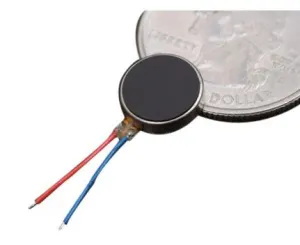Brown Dog Gadgets Solar Cockroach Vibrating Disc Motor
×10Resistor 1k ohm
×10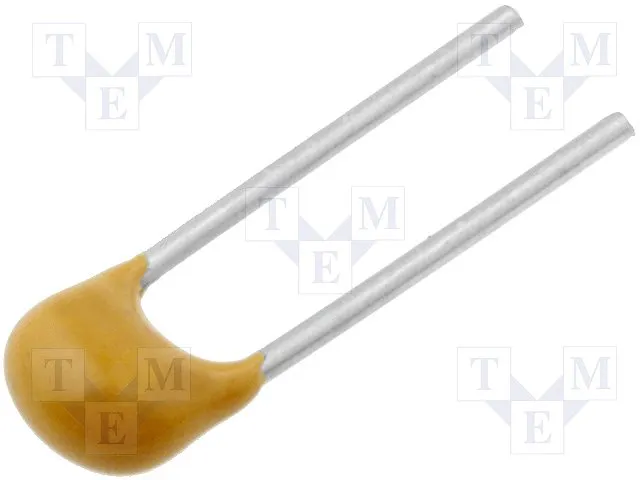Capacitor 100 nF
×10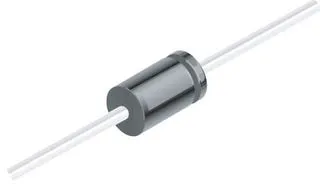1N4007 – High Voltage, High Current Rated Diode
×10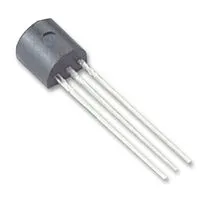General Purpose Transistor NPN
×10
 Switch regulator 3.3V
×1Arduino Nano R3
×1

### Software apps and online servicesArduino IDECycling '74 MAX

## Schematics

### Schematics

Ten small 3.3V motors controlled via Arduino.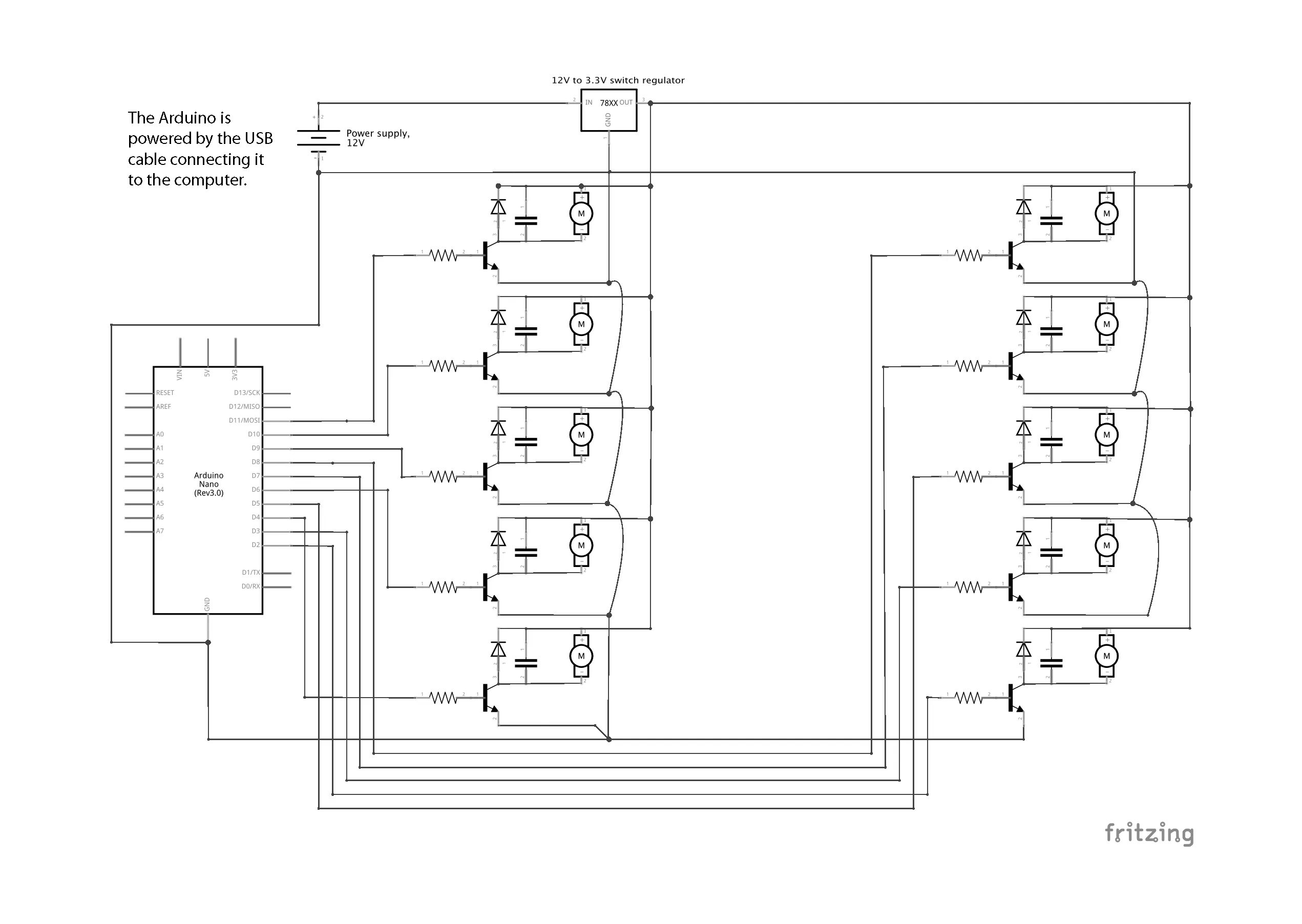## Code

### Code translating bytes to motor commands

Arduino
receiving a series of bytes and controlling ten motors via serial
```const byte numChars = 32;
byte CTL;
byte VAL;
const int motorPin1 = 11;   //PWM
const int motorPin2 = 10;   //PWM
const int motorPin3 = 9;    //PWM
const int motorPin4 = 6;    //PWM
const int motorPin5 = 4;          //ROTATING
const int motorPin6 = 8;
const int motorPin7 = 7;          //ROTATING
const int motorPin8 = 5;    //PWM
const int motorPin9 = 3;    //PWM
const int motorPin10 = 2;
boolean newData = false;

void setup() {
pinMode(motorPin1, OUTPUT);
pinMode(motorPin2, OUTPUT);
pinMode(motorPin3, OUTPUT);
pinMode(motorPin4, OUTPUT);
pinMode(motorPin5, OUTPUT);
pinMode(motorPin6, OUTPUT);
pinMode(motorPin7, OUTPUT);
pinMode(motorPin8, OUTPUT);
pinMode(motorPin9, OUTPUT);
pinMode(motorPin10, OUTPUT);

setPwmFrequency(3, 1);
setPwmFrequency(5, 1);
setPwmFrequency(6, 1);
setPwmFrequency(9, 1);
setPwmFrequency(10, 1);
setPwmFrequency(11, 1);

Serial.begin(38400);
}

void loop() {
recvWithStartEndMarkers();
writeToMotors();
showNewData();
}

void recvWithStartEndMarkers() {
static boolean recvInProgress = false;
static byte ndx = 0;
byte startMarker = 255;
byte endMarker = 250;
byte rc;

// if (Serial.available() > 0) {
while (Serial.available() > 0 && newData == false) {

if (recvInProgress == true) {
if (rc != endMarker) {
ndx++;
if (ndx >= numChars) {
ndx = numChars - 1;
}
}
else {
receivedChars[ndx] = '\0'; // terminate the string
recvInProgress = false;
ndx = 0;
newData = true;
}
}

else if (rc == startMarker) {
recvInProgress = true;
}
}
}

void showNewData() {
if (newData == true) {

Serial.print(" CTL ");
Serial.print(CTL);
Serial.print(" VAL ");
Serial.println(VAL);
newData = false;
}
}

void writeToMotors() {
if (newData == true) {

if (CTL == 1) {
analogWrite(motorPin1, VAL);
}
if (CTL == 2) {
analogWrite(motorPin2, VAL);
}
if (CTL == 3) {
analogWrite(motorPin3, VAL);
}
if (CTL == 4) {
analogWrite(motorPin4, VAL);
}
if (CTL == 5) {
if (VAL > 64) {
digitalWrite(motorPin5, HIGH);
}
else {
digitalWrite(motorPin5, LOW);
}
}

if (CTL == 6) {
if (VAL > 64) {
digitalWrite(motorPin6, HIGH);
}
else {
digitalWrite(motorPin6, LOW);
}
}

if (CTL == 7) {
if (VAL > 64) {
digitalWrite(motorPin7, HIGH);
}
else {
digitalWrite(motorPin7, LOW);
}
}
if (CTL == 8) {
analogWrite(motorPin8, VAL);
}

if (CTL == 9) {
analogWrite(motorPin9, VAL);
}
if (CTL == 10) {
if (VAL > 64) {
digitalWrite(motorPin10, HIGH);
}
else {
digitalWrite(motorPin10, LOW);
}
}

}
}

void setPwmFrequency(int pin, int divisor) {
byte mode;
if (pin == 5 || pin == 6 || pin == 9 || pin == 10) {
switch (divisor) {
case 1: mode = 0x01; break;
case 8: mode = 0x02; break;
case 64: mode = 0x03; break;
case 256: mode = 0x04; break;
case 1024: mode = 0x05; break;
default: return;
}
if (pin == 5 || pin == 6) {
TCCR0B = TCCR0B & 0b11111000 | mode;
} else {
TCCR1B = TCCR1B & 0b11111000 | mode;
}
} else if (pin == 3 || pin == 11) {
switch (divisor) {
case 1: mode = 0x01; break;
case 8: mode = 0x02; break;
case 32: mode = 0x03; break;
case 64: mode = 0x04; break;
case 128: mode = 0x05; break;
case 256: mode = 0x06; break;
case 1024: mode = 0x07; break;
default: return;
}
TCCR2B = TCCR2B & 0b11111000 | mode;
}
}
```

## Credits

### touchmysound

6 projects • 42 followers
Alessandro Perini's artistic production ranges from audiovisual and light-based works to net-art, land-art and vibration-based works.